# Tridecagon facts for kids

Kids Encyclopedia Facts
(Redirected from Tridecagram)
Regular tridecagon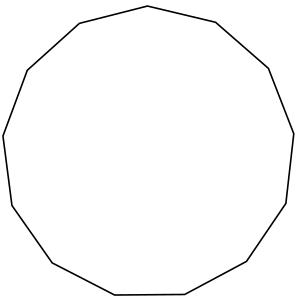A regular tridecagon
Type Regular polygon
Edges and vertices 13
Schläfli symbol {13}
Coxeter diagram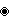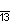Symmetry group Dihedral (D13), order 2×13
Internal angle (degrees) ≈152.308°
Dual polygon Self
Properties Convex, cyclic, equilateral, isogonal, isotoxal

A tridecagon or trikaidecagon or triskaidecagon or trisdecagon or 13-gon is a shape with 13 sides and 13 corners.

## Regular tridecagon

All sides of a regular tridecagon are the same length. Each corner is 147.27°. All corners added together equal 6840°.

### Area

The amount of space a regular tridecagon takes up is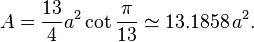$A = \frac{13}{4}a^2 \cot \frac{\pi}{13} \simeq 13.1858\,a^2.$

a is the length of one of its sides.

## Numismatic use

The regular tridecagon is used as the shape of the Czech 20 korun coin.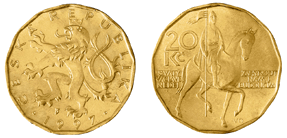## Images for kidsTridecagon Facts for Kids. Kiddle Encyclopedia.